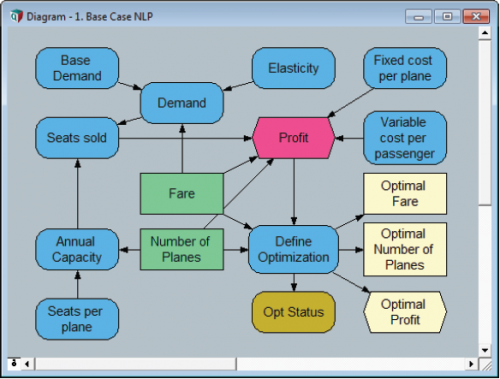# Airline NLP Module 1: Base Case

You can navigate to the Airline NLP example starting in the directory in which Analytica is installed: Example Models/Optimizer Examples/Airline NLP.ana.

The goal is to determine optimum ticket fare and the number of planes to operate such that the airline can achieve the highest profit possible.

Decisions are Fare and the Number of Planes. Since this is an NLP, the values we use to define these decisions will be used as initial guesses in the optimization. Fare will be a continuous variable with lower and upper bounds. Number of Planes will be an Integer value with lower and upper bounds.

Decision Fare := 200
Domain of Fare := Continuous(100, 400)
Decision Number_of_Planes := 3
Domain of Number_of_Planes := Integer(1, 5)


First, we assume a base level of demand in passenger-trips per year assuming a base fare of $200 per trip. Variable Base_Demand := 400k Actual demand will vary by price according to market demand elasticity. Variable Elasticity := 3 Variable Demand := Base_Demand*(Fare/200)^(-Elasticity) Each plane holds 200 passengers. We assume each plane makes two trips per day, 360 days per year. Variable Seats_per_plane := 200 Variable Annual_Capacity := Number_of_Planes*Seats_per_Plane*360*2 Annual seats sold is limited either by capacity or by passenger demand. Variable Seats_Sold := Min([Demand, Annual_Capacity]) We assume annual fixed cost per plane and variable cost per passenger. Variable Fixed_cost := 15M Variable Var_cost := 30 The objective, Profit, is the difference between revenue and cost. Objective Profit := Seats_sold*(Fare - Var_cost) - Number_of_Planes*Fixed_cost Finally, we create the optimization node and solution quantities. Variable Opt := DefineOptimization( Decisions: Number_of_Planes, Fare Maximize: Profit) Decision Optimal_Fare := OptSolution(Opt, Fare) Decision Optimal_Planes := OptSolution(Opt, Number_of_Planes) Objective Optimal_Profit := OptObjective(Opt) Variable Opt_Status := OptStatusText(Opt)The solution yields an optimal fare of$195 with three planes in service.